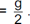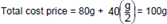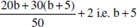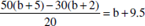### CAT 2017 – Slot 1 – Quantitative Ability – In a market, the price of medium quality mangoes is half that of good mangoes

Q. 1: In a market, the price of medium quality mangoes is half that of good mangoes. A shopkeeper buys 80 kg good mangoes and 40 kg medium quality mangoes from the market and then sells all these at a common price which is 10% less than the price at which he bought the good ones. His overall profit is
A) 6%
B) 8%
C) 10%
D) 12%

Explanation:- Let the price of each good mango be g.

Price of each medium quality mangoTotal selling price = 120(0.9g) = 108g Overall profit = 8%

##### Q. 2: If Fatima sells 60 identical toys at a 40% discount on the printed price, then she makes 20% profit. Ten of these toys are  destroyed in fire. While selling the rest, how much discount should be given on the printed price so that she can make the same amount of profit?A) 30% B) 25% C) 24% D) 28%

Correct Answer:- D Explanation:- Let the printed price be p.

If 40% discount is given, selling price = 0.6(60p) =36p

In order to make a profit of 20%, the selling price

Total cost price

=>36p/1.2 = 30p

Ten toys are destroyed in the fire.

The remaining toys are sold at a price such that the same amount of profit is made as in the conditional case. Profit made on remaining toys = 6p

Total selling price of remaining toys = 36p Discount that should be given = 50p – 36p = 14p Discount% = 28%

Q. 3: If a and b are integers of opposite signs such that (a + 3)^2 : b^2 = 9 : 1 and (a – 1)^2 : (b – 1)^2 = 4 : 1, then the ratio a^2 : b^2 is
A) 9:4 ‘
B) 81:4
C) 1: 4
D) 25: 4

We get 4 cases

CASE – 1

a+3 = 3b

a-1 = 2b-2

CASE – 2

a+3 = 3b

a-1 = 2b + 2

CASE – 3

a+3 = -3b

a-1 = 2b-2

CASE – 4

a+3 = -3b

a-1 = -2b+2

Subtracting the second equation from the first we get,

Case 1: 4 = b+2 => b = 2, a = 3 (Rejected as a and b should be of opposite sign)

Case 2: 4 = b-2 => b = 6, a = 15 (Rejected as a and b should be of opposite sign)

Case 3: 4 = -5b+2 => b = -2/5 (Rejected as both a and b are integers)

Case 4: 4 = -b-2 => b = -6, a = 15 (Accepted)

So, a^2/b^2 = (15/6)^2 = 25/4

Option (D)

Q. 4: A class consists of 20 boys and 30 girls. In the mid-semester examination, the average score of the girls was 5 higher than that    of the boys. In the final exam, however, the average score of the girls dropped by 3 while the average score of the entire class increased by 2. The increase in the average score of the boys is
A) 9.5
B) 10
C) 4.5
D) 6

Explanation:- Let the average score of the boys in the midsemester examination be b. Average score of the girls = b + 5

In the final exam, average score of the girls = b + 5 – 3 = b + 2.

Average score of the entire class increased by 2 and is henceAverage score of the boysIncreases in the average of boys is 9.5.

Q. 5: The area of the closed region bounded by the equation | x | + | y | = 2 in the two-dimensional plane is
A) 4π
B) 4
C) 8
D) 2π

Remember the formula |x| + |y| = n

Here, area bounded by the region = 2n^2

In the question, n=2

So, area = 8

Option (C)

##### Checkout Other Questions of CAT 2017 Slot 1 Paper:

Verbal Ability :              |   Q.01- Q.06  |  Q.07- Q.12  |  Q.13- Q.18  |  Q.19- Q.21  |  Q.22- Q.24  |  Q.25- Q.29  |  Q.30 – Q.34  |

Logical Reasoning :    |   Q.29 – Q.32  |

Quantitative Aptitude: |